### Temperature Sensors

Temperature sensors are among the most commonly used sensors. All types of equipment use temperature sensors, ranging from computers, cars, kitchen appliances, air conditioners, and (of course) home thermostats. The five most common types of temperature sensors include:
• Thermistor
• Thermocouple
• RTDs (resistive temperature detectors)
• Digital thermometer ICs
• Analog thermometer ICs
This article will give you a succinct intro to each of the sensor types listed.

### Thermistor

As the name implies, the thermistor (i.e., thermal resistor) is a temperature-sensing device whose resistance is a function of its temperature. Thermistors are available in two types: PTC (positive temperature coefficient) and NTC (negative temperature coefficient). The resistance of a PTC thermistor increases as the temperature increases. In contrast, the resistance of an NTC thermistor decreases as temperature increases, and this type seems to be the most commonly used thermistor. See Figure 1 below.   [caption id="" align="alignnone" width="608"]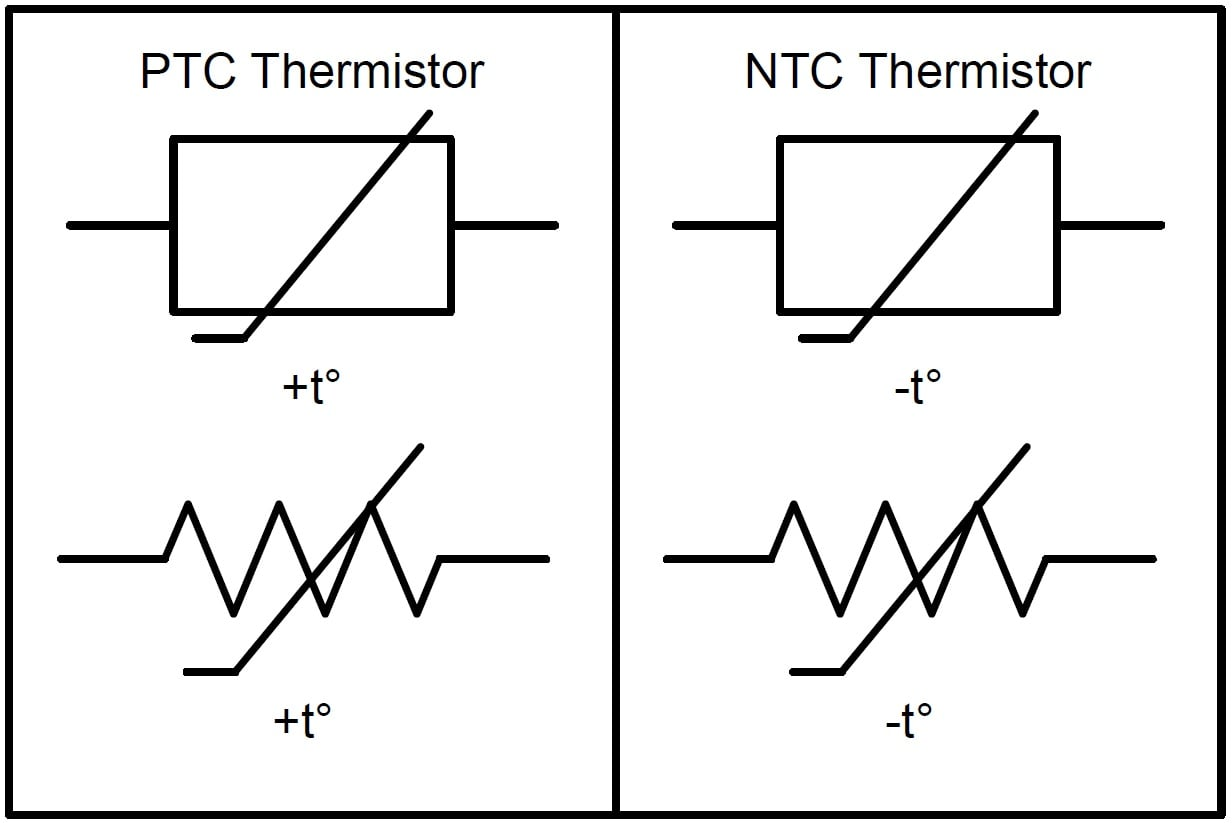Figure 1. PTC and NTC thermistor electrical symbols.[/caption]   It’s important to realize that the relationship between a thermistor’s resistance and its temperature is very non-linear. See Figure 2 below.   [caption id="" align="alignnone" width="500"]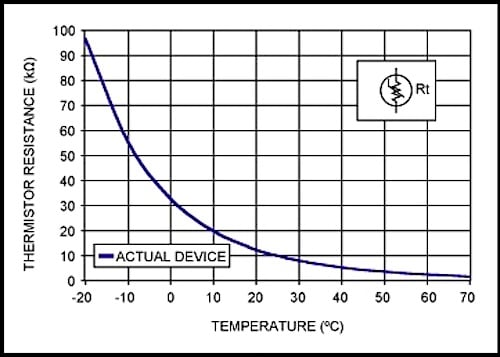Figure 2. An NTC thermistor resistance vs. temperature. Image courtesy of Maxim Integrated.[/caption]   The standard equation for an NTC thermistor’s resistance as a function of temperature is given by:
RT=R25Ce{β[(1/(T+273))(1/298)]}RT=R25C⋅e{β[(1/(T+273))−(1/298)]}
where
• R25C is the thermistor’s nominal resistance at room temperature (25°C). This value is normally provided in the datasheet.
• β (beta) is the thermistor’s material constant in Kelvin. This value is normally provided in the datasheet.
• T is the thermistor’s actual temperature in Celsius.
However, there are two easy techniques used to linearize a thermistor’s behavior, namely, resistive mode and voltage mode.

#### Resistive Mode Linearization

Resistive mode linearization places a normal resistor in parallel with the thermistor. If the value of the resistor is the same as that of the thermistor at room temperature, the region of linearization will be symmetrical around room temperature. See Figure 3 below.   [caption id="" align="alignnone" width="500"]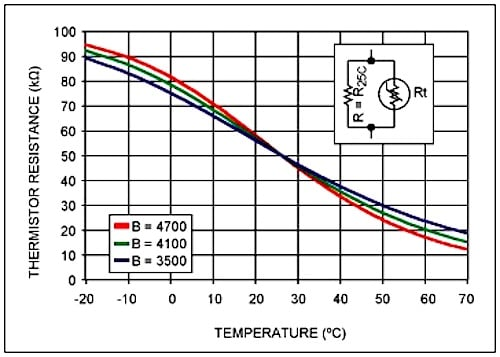Figure 3. Resistive mode linearization. Image courtesy of Maxim Integrated.[/caption]

#### Voltage Mode Linearization

Voltage mode linearization places the thermistor in series with a normal resistor forming a voltage divider circuit—the voltage divider circuit must be connected to a known, fixed, and stable voltage reference, VREF. This configuration has the effect of producing an output voltage that is somewhat linear over temperature. And, similar to resistive mode linearization, if the resistor’s value is equal to the thermistor’s resistance at room temperature, then the region of linearization will be symmetrical around room temperature. See Figure 4 below.   [caption id="" align="alignnone" width="500"]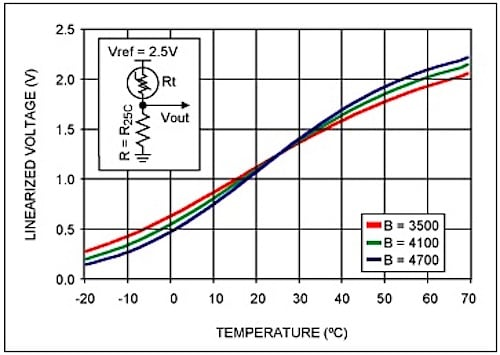Figure 4. Voltage mode linearization. Image courtesy of Maxim Integrated.[/caption]

### Thermocouple

Thermocouples are commonly used for measuring higher temperatures and larger temperature ranges. To summarize how thermocouples work, any conductor subjected to a thermal gradient will generate a small voltage. This phenomenon is known as the Seebeck effect. The magnitude of the generated voltage is dependent upon the type of metal. Practical applications of the Seebeck effect involve two dissimilar metals that are joined at one end and separated at the other end. The temperature of the junction can be determined via the voltage between the wires at the non-junction end. There are various types of thermocouples. Certain combinations of alloys have become popular and the desired combination is driven by variables including cost, availability, chemical properties, and stability. Different types are best suited for different applications, and they are commonly chosen based on the required temperature range and sensitivity. See Figure 5 for a graph of thermocouple characteristics.   [caption id="" align="alignnone" width="450"]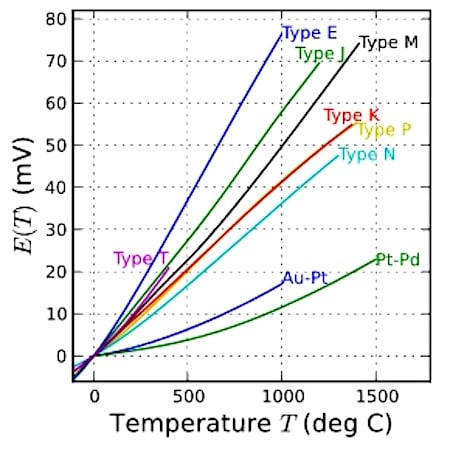Figure 5. Thermocouple characteristics. Image courtesy of Wikipedia.[/caption]

### Resistive Temperature Detectors (RTDs)

Resistive temperature detectors, also known as resistance thermometers, are perhaps the simplest temperature sensor to understand. RTDs are similar to thermistors in that their resistance changes with temperature. However, rather than using a special material that is sensitive to temperature changes—as with a thermistor—RTDs use a coil of wire wrapped around a core made from ceramic or glass. The RTD wire is of pure material, typically platinum, nickel, or copper, and the material has an accurate resistance–temperature relationship that is used to determine the measured temperature.

### Analog Thermometer ICs

Instead of using a thermistor along with a fixed-value resistor in a voltage divider circuit, an alternative solution would be an analog low-voltage temperature sensor, such as the TMP36 from Analog Devices. In contrast to a thermistor, this analog IC provides an output voltage that is almost linear; the slope is 10mV/°C over a temperature range of -40 to +125°C, and it’s accurate to ±2°C. See Figure 6 below.   [caption id="" align="alignnone" width="553"]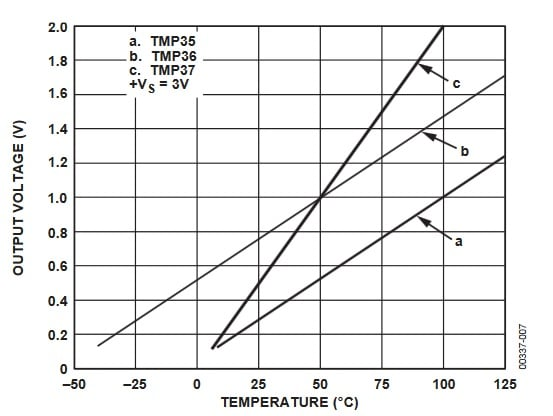Figure 6. Plot from the TMP36 datasheet.[/caption]   Although these devices are extremely easy to use, they are considerably more expensive than a thermistor-plus-resistor combination.

### Digital Thermometer ICs

Digital temperature devices are more complex, but they can be highly accurate. Also, they can simplify your overall design, because analog-to-digital conversion takes place inside the thermometer IC instead of a separate device such as a microcontroller. For example, the DS18B20 from Maxim Integrated has an accuracy of ±0.5°C and a temperature range of -55°C to +125°C. Also, some digital ICs can be configured to harvest energy from their data line, allowing them to be connected using just two wires (i.e., data/power and ground).     [caption id="" align="alignnone" width="922"]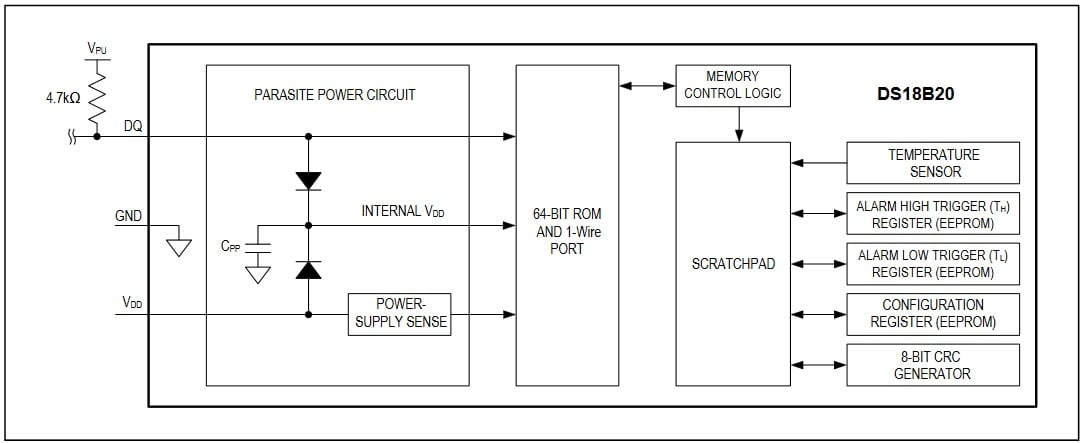Figure 7. DS18B20 block diagram, taken from the DS18B20 datasheet. Click to enlarge.[/caption]

### A Comparison between Types of Temperature Sensors

The table below shows a comparison between the different temperature sensors discussed. However, keep in mind that this information should be received as a generalization. The table is intended primarily for those who lack extensive experience with and/or knowledge of temperature sensors.   Table 1. A brief comparison of the temperature sensors discussed.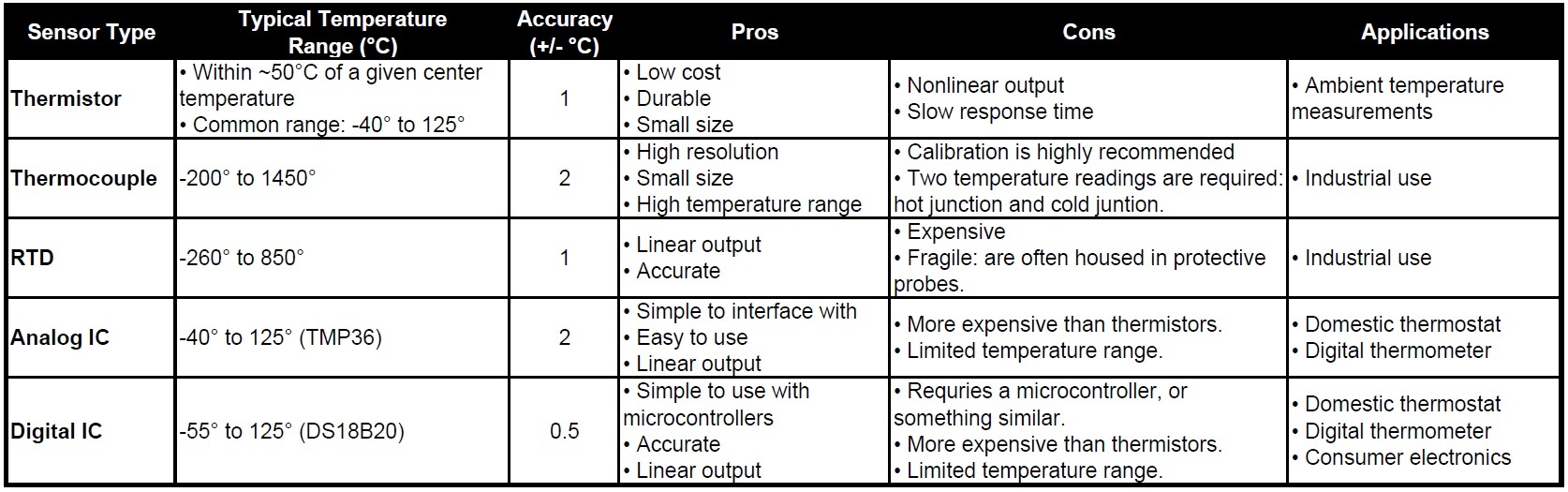Read More]]>